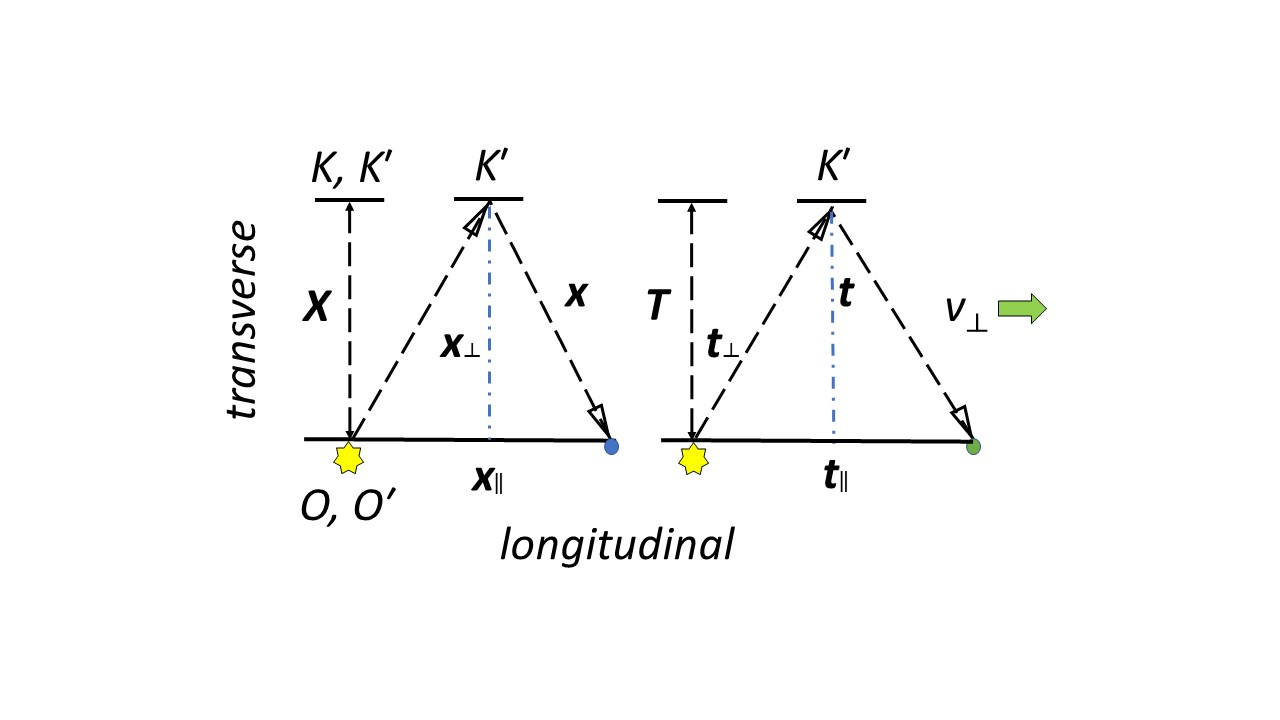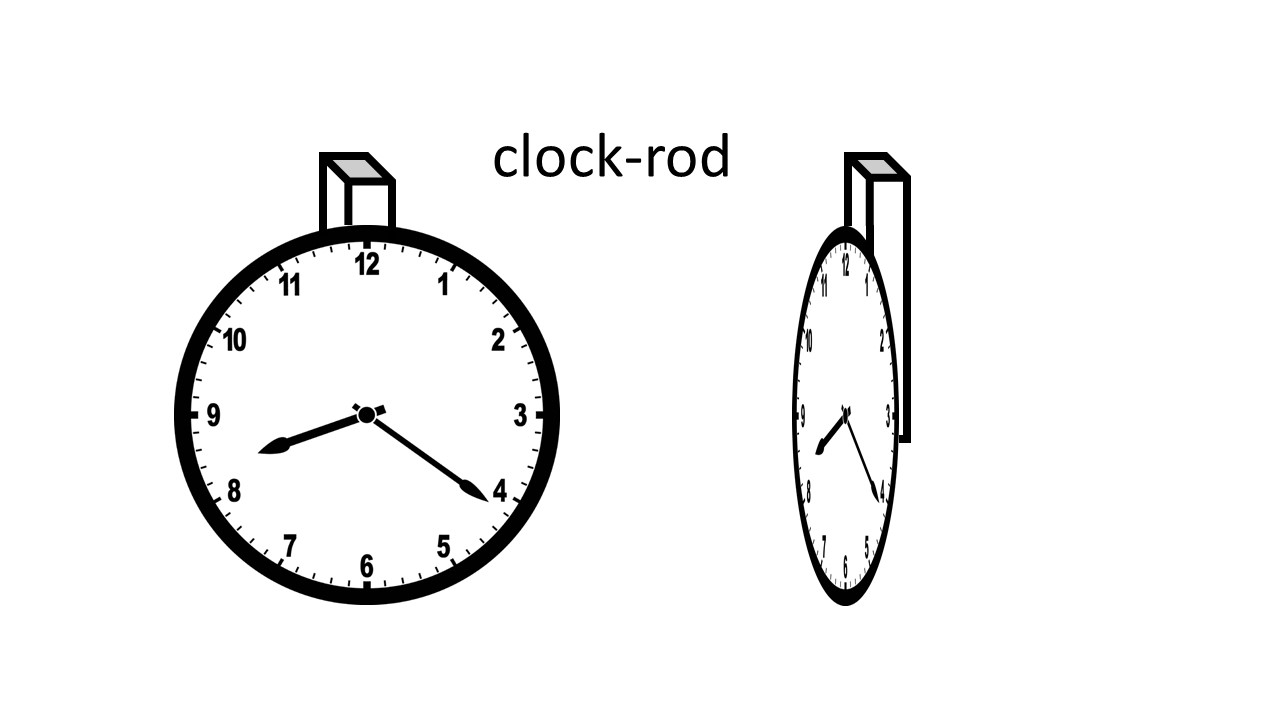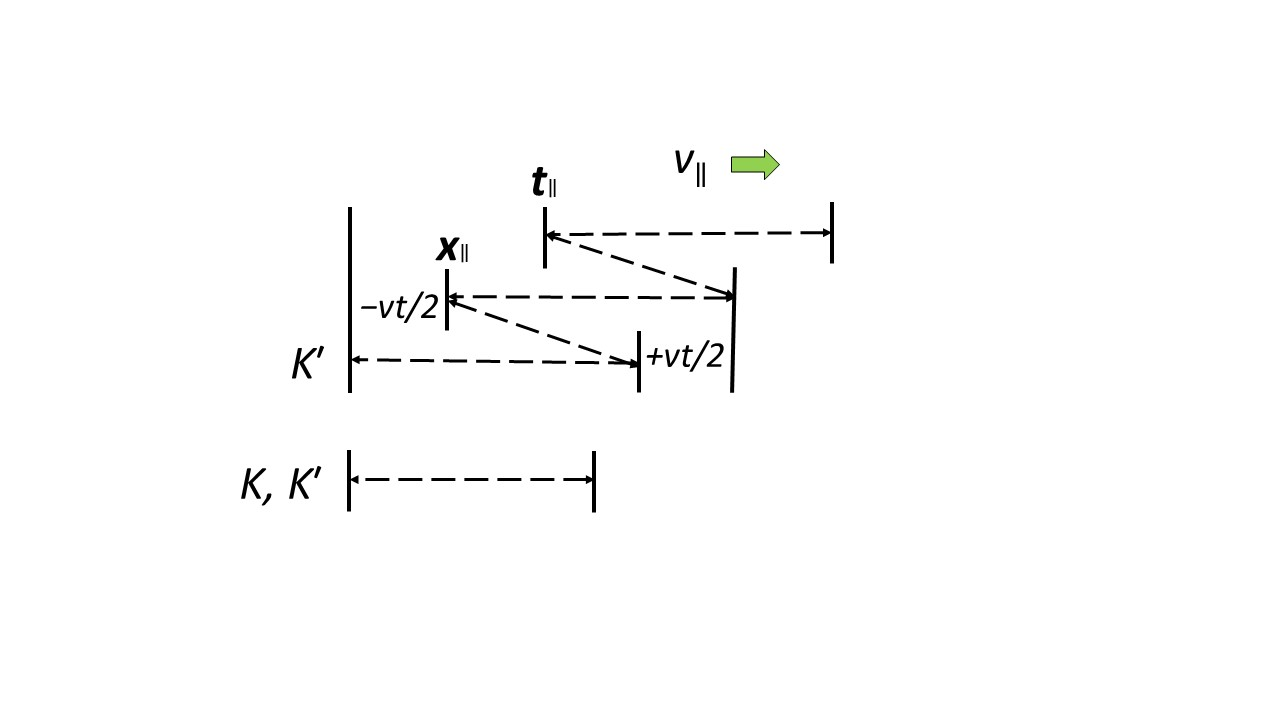iSoul In the beginning is reality.

# Harmonic conversion of space and time

As noted here, there are two kinds of mean rates: the time mean and the space mean. If the denominators have a common time interval, the time mean is the arithmetic mean and the space mean is the harmonic mean. If the denominators have a common space interval (stance), the space mean is the arithmetic mean and the time mean is the harmonic mean.

For example, light reflected back from a mirror at known distance forms two successive trips whose mean rate is the space mean pace. Several measurements with the same apparatus have a time mean pace. The mean speed is the inverse of the mean pace.

The general principle is that quantities with independent time (such as velocity) and a common time interval use ordinary algebra but such quantities with a common space interval use harmonic algebra. Alternately, quantities with independent space (stance) such as lenticity and a common space interval use ordinary algebra but such quantities with a common time interval use harmonic algebra.

In other words, quantities over the same interval with independent variables use ordinary algebra but quantities with different independent variables use harmonic algebra to convert between them.

For example, addition of velocities with a common time interval use ordinary vector addition (e.g., u + v) but addition of velocities with a common space interval use harmonic vector addition (e.g., ((1/u) + (1/v))−1 ≡ ((u+v)/u·v)−1 with u, v, u·v, u+v0.

The relativity parameter γ is based on a (3+1) spatial frame. The parameter γ in a temporal frame with a common time interval (k ≡ 1/c and ≡ 1/v) is:

γ² = (1 − v·v/c²)−1 ⇒ (1 − ℓ·ℓ/k²)−1.

The parameter γ in a temporal frame with a common space interval (stance) is:

γ² = (1 − v·v/c²)−1 ⇒ H(1 − ℓ·ℓ/k²)−1 = ((1 − k²/ℓ·ℓ)−1)−1 = (1 − k²/ℓ·ℓ) ≡ (1 − v·v/c²) = 1/γ².

# Interchanging space and time

The space-time exchange invariance, as stated by J. H. Field (see here) has an implicit second part. In addition to (1) the exchange (or interchange) of space and time coordinates, there is (2): the exchange of linear and harmonic algebra for ratios. Harmonic algebra is described here.

This is seen in the different averaging methods for velocities that differ spatially vs. velocities that differ temporally. If two vehicles take the same route, their average velocity is their arithmetic mean (u + v)/2, but if one vehicle has velocity u going and velocity v returning, then the average velocity is their harmonic mean 2/(1/u + 1/v). However, if one vehicle has pace u going and pace v returning, then the average pace is their arithmetic mean, but if two vehicles take the same route, their average pace is their harmonic mean.

Space and time are related to each other as covariant and contravariant components. If space is covariant, then time is contravariant, and if time is covariant, then space is contravariant.

The equations of space-time (3+1) and time-space (1+3) physics are symmetric to one another with the interchange of space and time dimensions. The equations of spacetime (4D) physics is self-symmetric. The interchange of space and time dimensions produces equivalent 4D equations.

To interchange the space and time coordinates, take these steps: For the equations of classical physics, (1) ensure either space or time is a parameter, (2) interchange one dimension with the parameter, and (3) expand the single dimension into three dimensions. For the equations of relativistic physics, (1) ensure there is a symmetry between space and time dimensions, (2) interchange one space and time dimension but leave dimensionless quantities unchanged, and (3) expand the single dimension into three dimensions.

These steps reflect the difference between Galileo’s and Einstein’s relativity. Galileo transforms one frame into another frame but does not combine frames as Einstein’s does. For example, Einstein requires all frames to have the same orientation, but Galileo accepts frame-specific orientations such as the right-hand rule.

The Galilean transformation represents the addition and subtraction of velocities as vectors. The dual Galilean transformation represents the addition and subtraction of lenticities as vectors. The Lorentz transformation represents the combination of Galilean and dual-Galilean transformations, as previously shown.

# One and two-way transformations

The transformation of Galileo is a one-way transformation, i.e., it uses only the one-way speed of light, which for simplicity is assumed to be instantaneous. The transformation of Lorentz is a the two-way transformation, which uses the universal two-way speed of light. The following approach defines two different one-way transformations, which combine to equal the two-way Lorentz transformation. Note that β = v/c; 1/γ² = 1 − β²; and γ = 1/γ + β²γ.

Galilean transformation:

Dual Galilean transformation:

These could be combined with a selection factor κ of zero or one:

Lorentz transformation (boost): .

General Lorentz boost (see here):

with   and k = 1/c for the Lorentz boost.

General dual Lorentz boost:

with and k = 1/c.

# Invariant intervals

Let’s begin with the space-time invariant interval r´² − ct´² = r² − ct². Then let us solve the equations:

r´ = Ar + Bt and t´ = Cr + Dt.

r´ = 0 = Ar + Btr = −tB/A = vt where v = −B/A {or} B = −Av

r´ = Ar + Bt = A(rvt)

A²(rvt)² − c²(Cr + Dt)² = r² − c²t²

A²r² − 2A²vrt + A²v²t² − C²c²r² − 2CDc²rtD²c²t² = r² − c²t²

⇒ (A² − C²c²)r² = r² {or} A² − c²C² = 1

⇒ (A²v² − D²c²)t² = −c²t² {or} D²c² − A²v² = c²

⇒ (2A²v + 2CDc²)rt = 0 {or} CDc² = −A²v

# 2D light clock

The famous Michelson-Morley experiment used what could be described as a 2D light clock since their interferometer combined two light clocks at right angles. Their hypothesis was that this would show the Earth moving through the aether, but they failed to detect any motion. Einstein explained this failure as a feature of relativity. In other words, the expected difference between the two light clocks was “corrected” by relativity.

The reasoning of the Michelson-Morley experiment went like this:

Light is sent from the source and propagates with the speed of light c in the aether. It passes through the half-silvered mirror at the origin at T = 0. The reflecting mirror is at that moment at distance L (the length of the interferometer arm) and is moving with velocity v. The beam hits the mirror at time T1 and thus travels the distance cT1. At this time, the mirror has traveled the distance vT1. Thus cT1 = L + vT1 and consequently the travel time T1 = L / (cv). The same consideration applies to the backward journey, with the sign of v reversed, resulting in cT2 = LvT2 and so T2 = L / (c + v). The longitudinal travel time T|| = T1 + T2 is:# Harmonic algebra

This revised post follows up on harmonic addition mentioned in a previous post here.

Harmonic algebra is an inverse algebra. It is based on an automorphism that interchanges the zero with the infinite and the greater-than-one with the less-than-one: 0 ↔ ∞ and x ↔ 1/x. So zero becomes the new inaccessible number and infinity becomes the new additive unit. That is,

xy := g−1(g(x) + g(y))

xy := g−1(g(x) ∙ g(y))

etc., where g(x) = 1/x with x ≠ 0.

Regular and harmonic algebra are isomorphic with ordinary addition exchanged with harmonic multiplication and ordinary multiplication exchanged with harmonic division. Harmonic algebra is like counting down from infinity, in which an increment of one reduces the amount slightly.

Harmonic addition is defined as a power operation:

with the understanding that (1/0) → ∞ and (1/∞) → 0. An intermediate value may be zero but not a final value.

Simple harmonic addition is thus defined as:

# Lorentz in spacetime and timespace

This post builds on the previous posts here and here, and follows the approach of J.-M. Levy here.

First, consider the Lorentz transformation in spacetime along the x axis per Levy’s section III:

Let us now envision two frames in ‘standard configuration’ with having velocity v with respect to K and let x, t (resp. x´, t´) be the coordinates of event M in the two frames. Let O and be the spatial origins of the frames; O and cöıncide at time t = = 0.

Here comes the pretty argument: all we have to do is to express the relation

OM = OO´ + O´M (equation 5)

between vectors (which here reduce to oriented segments) in both frames.

In K, OM = x, OO´ = vt and O´M seen from K is /γ since is O′M as measured in . Hence a first relation is:

x = vt + /γ (equation 6)

In , OM = x/γ since x is OM as measured in K, OO′ = vt´ and O′M = . Hence a second relation:

x/γ = vt´+ x´ (equation 7)

# Light clocks with multidimensional time

A previous post on this subject is here. One reference for this post is V. A. Ugarov’s Special Theory of Relativity (Mir, 1979).

A light clock is a device with an emission-reflection-reception cycle of light that registers the current timeline point and placeline point in units of cycle length and duration. Consider two identical light clocks, at first in their reference frames at rest, K, K´. Then, as the light clock in K´ moves transversely relative to K with uniform motion at velocity v (right), from K one observes the following:The illustration above shows one cycle length of the light path (i.e., wavelength), X, on the left and one cycle duration (i.e., period), T, on the right at rest in reference frames K, K´. For the reference frame K´, in motion relative to reference frame K, call the arc length of one cycle of the light path x. Call the distance between the beginning and ending place points of one cycle x. For the reference frame K´ relative to reference frame K, call the arc time of one cycle of the light path t. Call the distime between the beginning and ending timepoints of one cycle t.

# Clock-rods

A clock-rod is a linear or planar clock with a parallel rod attached to it. A mechanical or electronic clock-rod might look like this:Three clock-rods mutually perpendicular would measure length and duration in all directions.

A light clock-rod is conceptually like this:The clock and rod are parallel to each other so that parallel or perpendicular motion would change either the measurements of either the clock or the rod but not both. A complete harmonic cycle is not affected by motion:The +vt/2 increased distance of the first half-cycle is offset by the −vt/2 decreased distance of the second half-cycle. Likewise for the times +2d/v and −2d/v.

# Kinematic proofs

Displacement with distime: displacement s, distime (time interval) t, velocities v1 and v, acceleration a:

To prove: v = v1 + at

a = (vv1) / t    by definition

at = (vv1)     multiply by t

v = v1 + at

To prove: s = v1t + ½ at2

vavg = s / t          by definition

vavg = (v1 + v) / 2        by definition

s / t = (v1 + v) / 2       combining these two

s = (v1 + v) t / 2         multiply by t

s = (v1 + (v1 + at)) t / 2       from above

s = (2v1 + at) t / 2

s = v1t + ½ at2